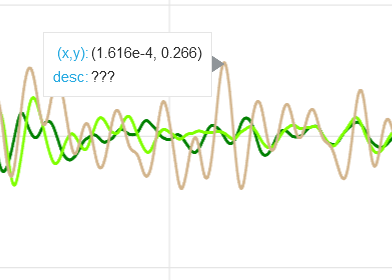# How to add hovetool to multiple line plot

Hello everybody,

I have multiple data files that each contain x and y. I’ve tried to plot these files on the same figure but they would be overlapping due to proximity of y values between these files. Thus, I’ve created a new column for each of the data files to cascade the y values. Then I would plot the x, and the cascaded y-values to offset individual charts instead of overlapping them.

This would result in an incorrect y-value for each file; which is acceptable. However, I want to show the original y-value before offsetting using hovertool. I tried my hands to at it; alas, it’s not working.

``````
from bokeh.layouts import layout
from bokeh.models import ColorPicker, RangeSlider,HoverTool
from bokeh.plotting import Figure, output_file, show

TOOLTIPS = [
("t", "\$x"),
("A", "@A"),
]

p = Figure(
x_range=(dfs['t'].min(), dfs['t'].max()),
x_axis_label = "t (ns)",
y_axis_label = "mV",
plot_width=850,
plot_height=600

)
line = p.line(x='t',legend_label=file_names[i],
color=random.choice(color),
source = source[i],
line_width=2)
hover_tool = HoverTool(tooltips=TOOLTIPS)
hover_tool.mode = 'mouse'
p.legend.location = "top_left"
p.legend.click_policy="hide"

output_file("test.html", title="test")

range_slider = RangeSlider(
start=0,
end=dfs['t'].max(),
step=1e-5,
value=(p.x_range.start, p.x_range.end),
)
range_slider.format = "0.000000"

##Final Chart layout
layout = layout([
[range_slider],
[p]])

show(layout)
``````

I’m getting the following error:
`RuntimeError: Models must be owned by only a single document, ColumnDataSource(id='1002', ...) is already in a doc`

I’m afraid I don’t really understand what this means at all, either what you are trying to accomplish, or what is happening that is different from what you want to happen. Screenshots, sketch diagrams, etc could be helpful here.

As for the other, the code above is not runnable (`dfs` is not defined). Please update the code to be an actual complete, runnable Minimal Reproducible Example so that it can be investigated directly. Otherwise, all I can note is that in general that error happens if you try to re-use Bokeh objects across different calls to `show`. Either don’t do that (best practice) or call `reset_output` manually.

Lastly, please use `figure` instead of `Figure`. We have only ever demonstrated use of `figure` and `Figure` (capital-F) will be going away in Bokeh 3 later this year.

Thank you for allocating the time to reply to my post.

This is what is originally plotted.Then I multiply the Y-value for each line to get the following offset between individual lines

Please find below a Minimal Reproducible Example:

``````
import pandas as pd
import numpy as np
import random
from bokeh.layouts import layout
from bokeh.models import ColorPicker, RangeSlider,HoverTool,ColumnDataSource
from bokeh.plotting import figure, output_file, show

x = np.linspace(0,0.01,100)
y1 = np.linspace(random.uniform(-1, 1),random.uniform(-1, 1),100)
y2 = np.linspace(random.uniform(-1, 1),random.uniform(-1, 1),100)
y3 = np.linspace(random.uniform(-1, 1),random.uniform(-1, 1),100)
y4 = np.linspace(random.uniform(-1, 1),random.uniform(-1, 1),100)

dfs = {0:[x,y1],
1:[x,y2],
2:[x,y3],
3:[x,y4]}

fixed_offset = {}
source={}

for i in range(4):
dfs[i] = pd.DataFrame(dfs[i]).T
dfs[i].columns = ['t','A']

for u in range(4):
fixed_offset[u] = dfs[u].max().shift(1).fillna(0).cumsum() + [i*cascade[u] for i in range(dfs[u].shape)]
source[u] = ColumnDataSource(dfs[u])

color=['blue',
'green',
'black',
'red',
'darkviolet']

TOOLTIPS = [
("(x,y)", "(\$x, \$y)"),
("desc", "@A"),
]

p = figure(
x_range=(dfs['t'].min(), dfs['t'].max()),
x_axis_label = "t (ns)",
y_axis_label = "mV",
plot_width=850,
plot_height=600

)
for i in range(4):
line = p.line(x='t',
color=random.choice(color),
source=source[i],
line_width=2)

hover_tool = HoverTool(tooltips=TOOLTIPS)
hover_tool.mode = 'mouse'
p.legend.location = "top_left"
p.legend.click_policy="hide"

output_file("Test.html", title="Test")

range_slider = RangeSlider(
start=0,
end=dfs['t'].max(),
step=1e-5,
value=(p.x_range.start, p.x_range.end),
)
range_slider.format = "0.000000"

##Final Chart layout
layout = layout(
[

[range_slider],
[p],
]
)
show(layout)
``````

I’m now getting the following error:

`TypeError: 'Column' object is not callable`

How can I

``````reset_output
``````

manually?

This doesn’t work

``````from bokeh.plotting import reset_output

reset_output()
``````

thank you @Bryan

2 Likes

This topic was automatically closed 90 days after the last reply. New replies are no longer allowed.